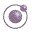1 minute, 19 seconds Higher-order Functions - slide 32 : 34

The function simple-html-table

In an earlier exercise - 'restructuring of lists' - we have used the function simple-html-table

We will now show how it can be implemented

```(define simple-html-table
(lambda (column-widht list-of-rows)
(let ((gmap (curry-generalized map))
(td-width
(modify-element td 'width
(as-string column-widht))))
(table
'border 1
(tbody
(gmap (compose tr (gmap td-width)) list-of-rows))))))```